Questions from the forum
 Q: Anybody knows what the lite-C equivalent of "my.oriented = on;" is? A: Use "set(my, DECAL);" for any sprite that's applied over a wall as a painting, etc. If both sides of the sprite have to be visible, simply change its pan or tilt angles to a tiny non-zero value (such as 0.1 or so).     Q: I'm trying to make a text zone, using a panel as background, and a text to show some text lines, using a ttf font. I want to show the text with "hyperlinks", that is, if the text is black over a white background, some of the text words are highlighted on blue, and so on. I think that the way to develop this is by using more text controls, depending of the number of words or lines to show. Is there an easier way to achieve this? A: Here's an example that creates texts at runtime and allows you to specify their position and colors:   TEXT* temp_txt;   STRING* temp_str = "#50";   FONT* arial_font = "Arial#20";   function colored_texts(VECTOR* text_color, VECTOR* text_position) {        temp_txt = txt_create(1, 10); // create a text with a string and layer = 10        str_cpy((temp_txt.pstring), temp_str); // assign it the proper string        temp_txt.font = arial_font;        temp_txt.red = text_color.x; // set the color of the text        temp_txt.green = text_color.y;        temp_txt.blue = text_color.z;        temp_txt.pos_x = text_position.x; // set the position of the text (the z component of the vector isn't used)        temp_txt.pos_y = text_position.y;        temp_txt.flags = SHOW; // now display the text }   function texts_startup() {        wait (-5); // wait a bit        str_cpy(temp_str, "Get ready!"); // set the first string        colored_texts(vector(100, 220, 130), vector(300, 200, 0)); // create the first text        wait (-2); // wait a bit        str_cpy(temp_str, "The level is being loaded"); // set the second string        colored_texts(vector(255, 20, 220), vector(100, 400, 0)); // create the second text        wait (-2); // wait a bit        str_cpy(temp_str, "Game over!"); // set the third string        colored_texts(vector(125, 120, 250), vector(500, 550, 0)); // create the third text        // and so on }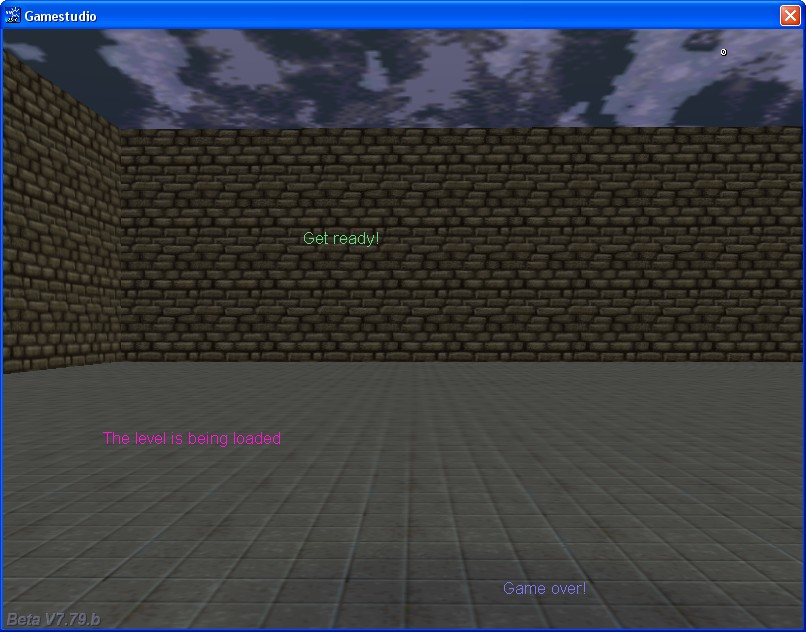Q: I'd like to get started on a simple 3D breakout game. Do you have a small sample? A: There you go:   #include #include   ENTITY* ball; // defined for further use   function control_ball();   function main() {        video_mode = 8; // 1024 x 768 pixels        video_screen = 1; // full screen mode        level_load("breaker.wmb");        while (!player) {wait (1);}        ball = ent_create("ball.mdl", vector (800, 0, -250), control_ball); }   action players_paddle() {        player = my;        set(my, TRANSLUCENT);        my.alpha = 70;        while (1)        {                c_move (my, nullvector, vector(0, -50 * mouse_force.x * time_step, 40 * mouse_force.y * time_step), NULL);                wait (1);        } }   function ball_was_hit() {        vec_to_angle(my.pan, bounce); // makes the ball bounce off on collisions        // add some randomness to the ball (we wouldn't want to see it bouncing back and forth between 2 obstacles)        my.pan += 1 - random(2); }   function control_ball() {        my.emask |= (ENABLE_IMPACT | ENABLE_ENTITY | ENABLE_BLOCK);        my.event = ball_was_hit;        while(1)        {                my.skill1 = c_move (my, vector(50 * time_step, 0, 0), nullvector, IGNORE_PASSABLE);                if (my.skill1 < 5) // the ball got stuck? might be needed under special circumstances                {                        my.pan = random(360); // then help it escape by setting a random pan angle for it!                }                wait(1);        } }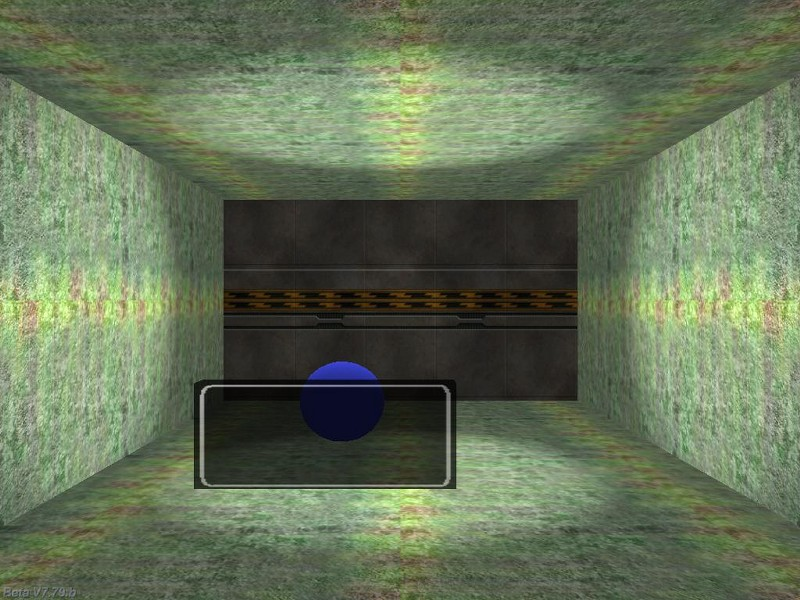Q: Does anyone have a script that allows me to play a movie on a surface in a level? I would like to be able to click the surface in order to replay it. A: Here's an example:   BMAP* pointer_tga = "pointer.tga";   function mouse_startup() {         mouse_mode = 2;        mouse_map = pointer_tga;        while (1)        {                 vec_set(mouse_pos, mouse_cursor);                wait(1);        } }   function surface_clicked() {        media_play ("mymovie.avi", bmap_for_entity (my, 0), 100); // use your own movie name }   // attach this action to a sprite or a model // if you are using a sprite, make sure that it has the same size (in pixels) with the size of the movie action movie_surface() {        my.emask |= ENABLE_CLICK;        my.event = surface_clicked;        while (!player) {wait (1);} // wait until the player model is loaded        wait (-10); // wait for 10 seconds, and then play the movie once        media_play ("mymovie.avi", bmap_for_entity (my, 0), 100); // use your own movie name }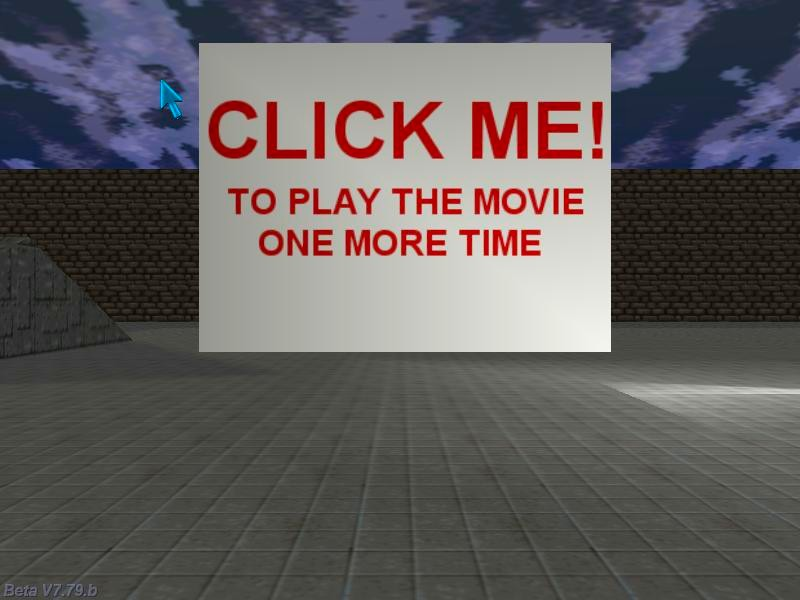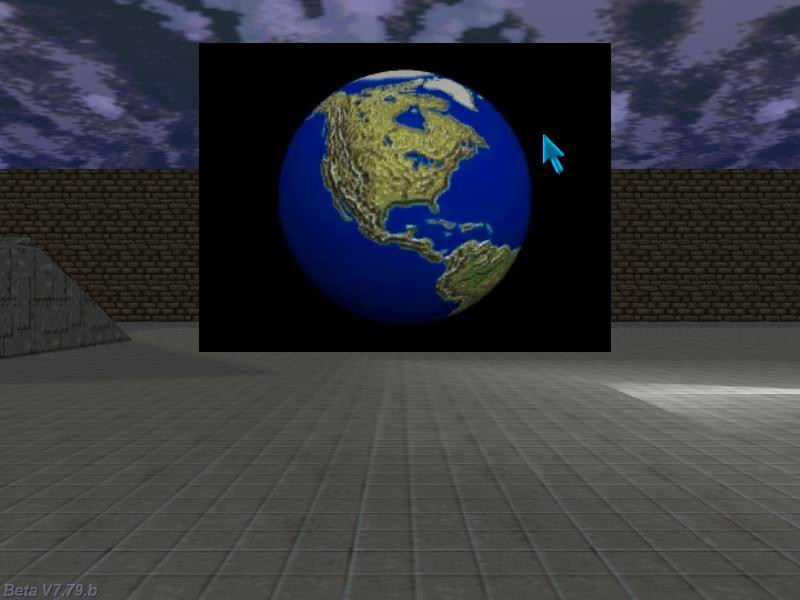Q: I am using the variable "PI" in my Lite-C code but get an error that says it is "undeclared identifier". Maybe I am missing some "include" to be able to use it? A: PI isn't a keyword in lite-C, but you can easily define it using a single line of code that produces a very good approximation:   #define PI 355/113     Q: I'm having a problem with the menu code from Aum69. When I click on new game it gives me a notification "Empty prototype called in." The game starts and you can play it but you have to click on ok for the notice window to disappear. I would like to use this menu system as the base for my game but I have been unable to fix this notification problem. A: The new A7 engine versions can catch even tiny syntax errors; take a look at the lines of code below to see the correct function prototypes:   function mouse_over(); function plasma_jet(); function particle_fade(PARTICLE *); // this function prototype definition wasn't correct function particle_jet(PARTICLE *); function particle_jet_small(PARTICLE *); function robot_explodes();     Q: I want to make a tower defense game. How can I tell the tower to fire at the enemy with the smallest health value? A: Here's an example:   var minimum_health = 100;   ENTITY* turret; ENTITY* enemy_target;   action my_turret() // attach this action to your turret {        turret = my;        VECTOR temp;        while (1)        {                // scan all the entities that are closer than 1000 quants to this entity                c_scan(my.x, my.pan, vector(360, 180, 1000), IGNORE_ME);                  if (enemy_target) // an enemy target was detected?                {                        vec_set(temp, enemy_target.x);                        vec_sub(temp, my.x);                        vec_to_angle(my.pan, temp); // rotate towards the enemy target                        if (total_frames % 120 == 1) // fire a bullet every 2 seconds                        {                                // ent_create("bullet.mdl", my.x, move_bullet); // use your own shooting code here                        }                }                wait (1);        } }   function i_am_scanned() {        if (my.skill1 <= minimum_health)        {                minimum_health = my.skill1;                enemy_target = my;                my.scale_z = 1.5; // highlight the enemy with the minimum health value (make it taller)        }        else        {                my.scale_z = 1;        } }   action my_enemies() // attach this action to your enemies {        my.skill1 = random(100); // each enemy has a random health value        my.emask |= ENABLE_SCAN; // make the entity sensitive to scanning        my.event = i_am_scanned; }   function init_startup() {        fps_max = 60; // limit the frame rate to 60 fps        random_seed(0); // generate random values at each game run }     Q: I have a light switch, and somewhere else the actual light that needs to be turned on by that switch. How can I do that? A: Make the switch scan for the player; the code below turns on the light as soon as the player approaches the switch.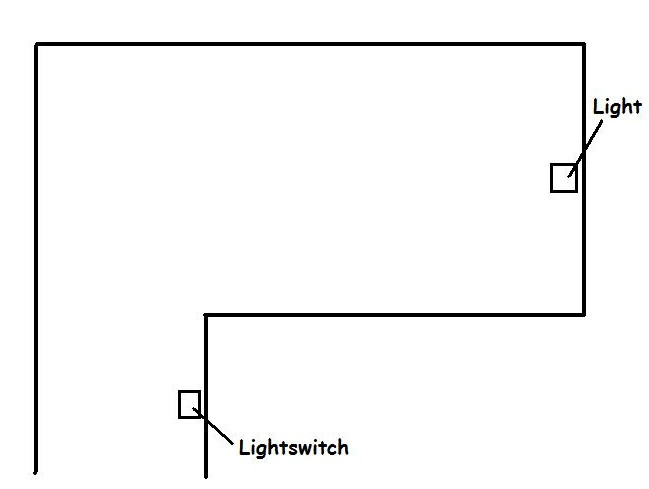var light_on = 0; // the light is turned off at first   action my_switch() // attach this action to your light switch {        while (1)        {                // scan the entities that are closer than 100 quants to the light switch                c_scan(my.x, my.pan, vector(360, 180, 100), IGNORE_ME);                if (you == player) // the player has come closer than 100 quants to the light switch entity?                {                        light_on = 1; // then turn the light on!                }                wait (1);        } }   action my_lightbulb() // attach this action to your light entity {        while (!light_on) {wait (1);} // wait until the player comes close to the switch        set (my, CAST);        my.lightrange = 500;        my.red = 200;        my.green = 200;        my.blue = 160;                 }     Q: If I create a string such as    STRING* MyString = "Hi out there";  is there a way I can retrieve individual characters such as the letter H, and then the letter i, etc? A: Use the following snippet:   var string_length, string_index;   STRING* MyString = "Hi out there"; STRING* temp_str = "#50"; // just a backup string STRING* output_str = " "; // displays the string character by character   TEXT* output_txt = {        pos_x = 200;        pos_y = 20;        string(output_str);        flags = SHOW; }   function chars_startup() {        string_index = 0;        while (!key_s) {wait (1);} // press the "S" key to start the action        while (key_s) {wait (1);} // wait until the "S" key is released        string_length = str_len(MyString); // get the length of the string        while (string_index < string_length) // go through all the characters of the string, one by one        {                str_cpy (temp_str, MyString); // first of all, copy MyString to temp_str (we don't want to destroy it)                str_clip(temp_str, string_index); // cut the needed number of characters from the beginning of the string                str_trunc(temp_str, string_length - string_index - 1); // and cut from the end as well                // here's we've got all the characters of the string rolling one by one, so let's display them                str_cpy(output_str, temp_str); // copy the truncated temp_str to output_str             string_index += 1; // move on to the following character in the string                wait (-1); // display a character each second        }        str_cpy(output_str, " "); // hide the last character after it has been displayed }       Q: Does anyone have a code snippet that would send one entity to the position of another entity, but also over shoot its position and correct itself? This way the entity would look like it's jiggling its position when it gets to its target position. A: Use this example as a base for your code:   ENTITY* my_target;   action my_destination() // attach this action to the target entity {        my_target = my;        }   action target_seeker() // attach this action to your target seeking entity {        while (!my_target) {wait (1);} // wait until the target entity is loaded        VECTOR temp, temp_angle;        var temp_time;        vec_set(temp_angle, my_target.x);          vec_sub(temp_angle, my.x);          vec_to_angle(my.pan, temp_angle);        my.tilt = 0;        // the entity was rotated towards the destination here        // let's change its angle a bit, making it miss the target at first        if (random(1) > 0.5)                my.pan += 5; // play with 5        else                my.pan -= 5; // play with -5        // we have added -5 or 5 degrees to the pan angle here, so the entity will miss the target a bit        // the following loop runs until the entity comes close enough to the target          while (vec_dist(my.x, my_target.x) > 100) // play with 100        {                c_move (my, vector(5 * time_step, 0, 0), nullvector, IGNORE_PASSABLE | GLIDE);                wait (1);        }        // keep the entity moving in the wrong direction for 2 more seconds        temp_time = 0;          while (temp_time < 2)        {                c_move (my, vector(5 * time_step, 0, 0), nullvector, IGNORE_PASSABLE | GLIDE);                temp_time += time_step / 16;                wait (1);        }        // now rotate towards the target correctly        vec_set(temp_angle, my_target.x);          vec_sub(temp_angle, my.x);          vec_to_angle(my.pan, temp_angle);          while (vec_dist(my.x, my_target.x) > 40) // stop when the entity is closer than 40 quants to the target (play with 40)        {                c_move (my, vector(5 * time_step, 0, 0), nullvector, IGNORE_PASSABLE | GLIDE);                wait (1);        }        // the goal was reached here }